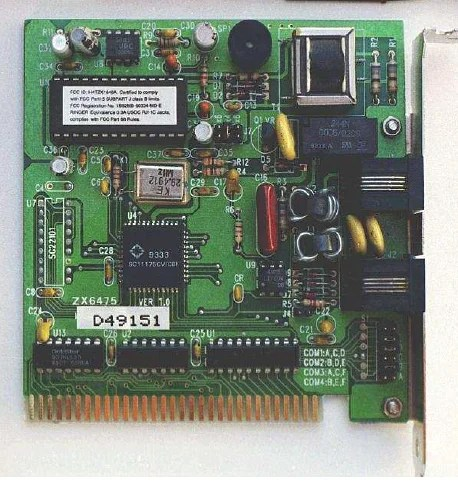# Wait some time if Book is not loaded…………….!

Electric Circuits Volume I – DC Handbook

This book is offering details as mentioned following chapter wise.

In First Chapter of this book offers BASIC CONCEPTS OF ELECTRICITY

In 2nd Chapter of this book includes information OHM’s LAW

In 3rd Chapter of this book covers ELECTRICAL SAFETY

In 4th Chapter of this book is comprehensive guide to SCIENTIFIC NOTATION AND METRIC PREFIXES

In 5th Chapter of this book describes SERIES AND PARALLEL CIRCUITS

In 6th Chapter of this book develops DIVIDER CIRCUITS AND KIRCHHOFF’S LAWS

In 7th chapter of this is discussing about SERIES-PARALLEL COMBINATION CIRCUITS

In 8th chapter of this book gives information about DC METERING CIRCUITS

In 9th chapter of this book illustrates about ELECTRICAL INSTRUMENTATION SIGNALS

In 10th chapter of this book explains about NETWORK ANALYSIS, BATTERIES AND POWER SYSTEMS, PHYSICS OF CONDUCTORS AND INSULATORS, CAPACITORS, MAGNETISM AND ELECTROMAGNETISM, INDUCTORS, RC AND L/R TIME CONSTANTS